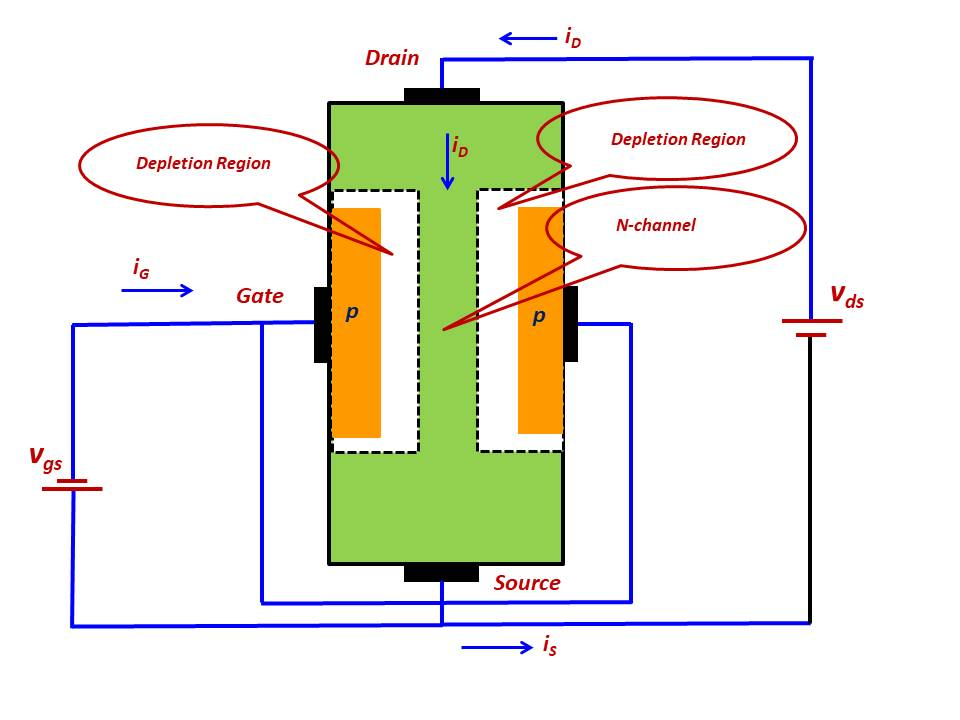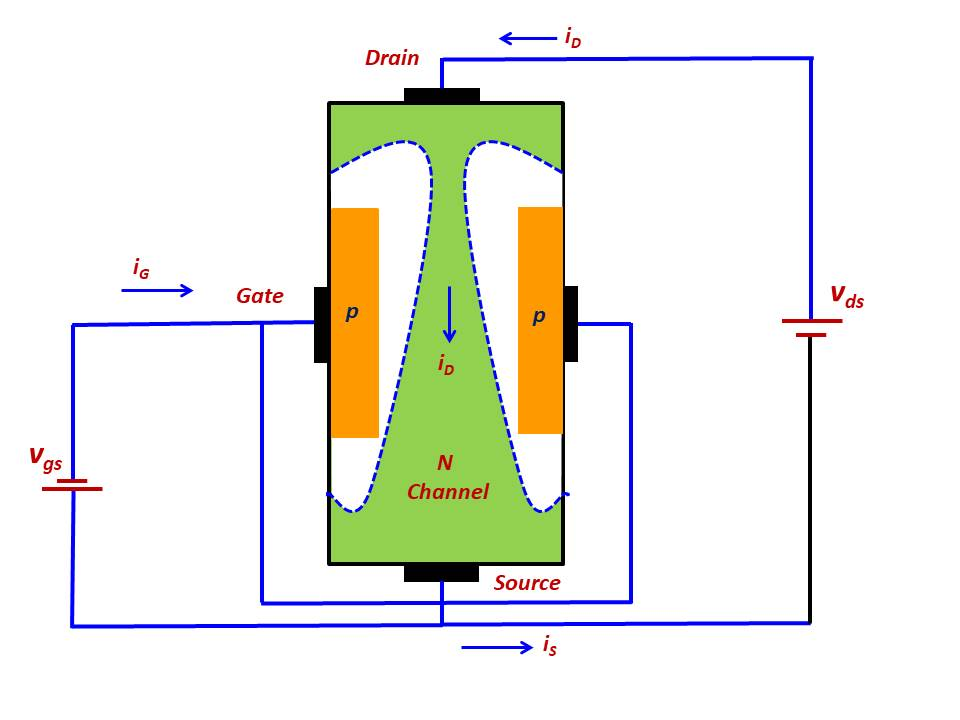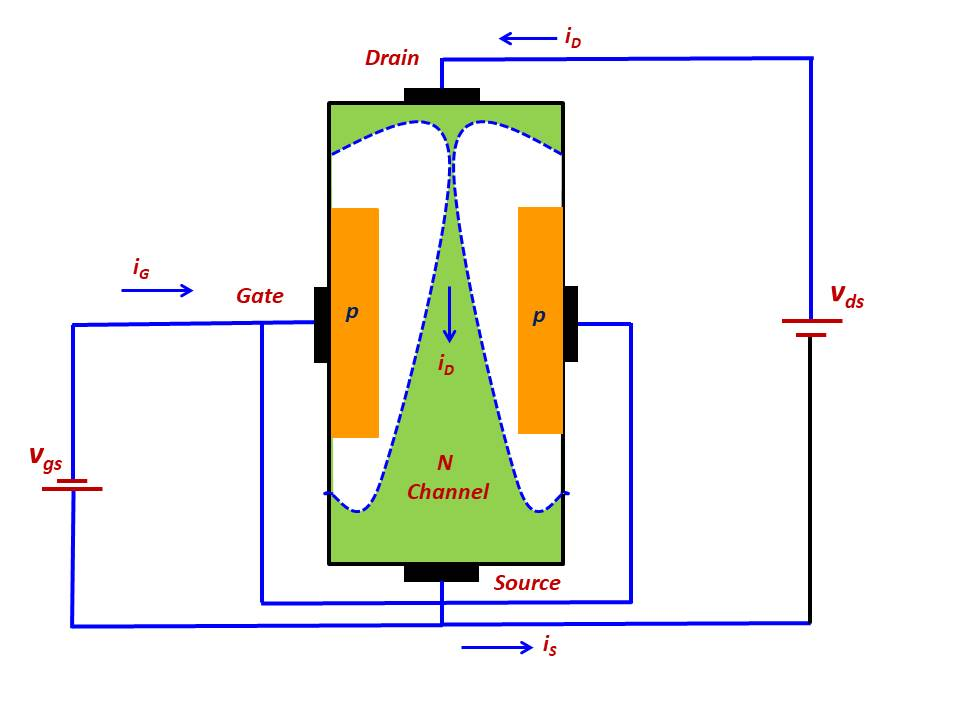### N Channel JFET

The Device structure of the N-Channel JFET is shown in Figure 3.As seen from the Figure 3 above, the structure of n-channel JFET consists of n type substrate the two sides of which are diffused with p type material. The n region is called the Channel as it forms a path between the Source and Drain. The two p - regions are electrically connected together and the common terminal is called Gate.

Note 1: For a p- channel JFET reverse all the semiconductor types i.e. n-channel is replaced with p-channel and p-type diffussions are replaced by n-type diffussions. Similarly the bias voltages are also reversed.

Note 2: The JFET is a symmetrical device i.e. the terminals Source and Drain are interchangeable. But it is useful to designate one terminal as Source and the other as Drain.

Why the name JFET?

The fundamental operation of the device depends on how the pn junction is biased. Here the junction is reverse biased and this controls the width of the channel and thus the current flow between Drain and Source. As seen the pn junction plays a key role in the operation of the device and hence the name Junction Field - Effect Transistor (JFET).

Working of the n-Channel JFET:

Figure 4 shows the electrical connections of the n-channel JFET.Figure 4: Electrical connections and direction of current flow

Let the voltage applied to the Gate terminal is 0 i.e. vgs=0 and the voltage vds is finite and the polarity is as shown in the Figure 4 above. Under these bias conditions there is a flow of current iD from Drain to Source.

Case 1: Now consider the case where the vgs become negative, the depletion region of the Gate - Channel spreads i.e. widens. This effectively reduces the channel i.e. the channel becomes narrower, thus increasing the channel resistance. Now because of this phenomenon the drain current iD decreases for a given Vds. Here the value of Vds is small and thus the channel maintains almost a uniform width. Here the JFET is operating like a resistance whose value is controlled by the Vgs. As the value of Vgs is made more and more negative, the depletion width increases and it occupies the entire channel. This will result in the channel being completely depleted of charge carriers i.e. electrons in an n-channel JFET. This situation may be now considered as the effective disappearance of the channel. It may be noted that the Gate voltage is negative for the n-channel JFET. This value of Vgs is called threshold voltage or pinch off voltage Vp in case of JFETs.

Case 2: Under this case keep the Gate - Source voltage Vgs constant and let its value be negative and less than the pinch off voltage and increase the voltage applied between Drain and Source Vds. The Vds appears as voltage drop across the length of the channel, and the voltage drop increases as we move along the channel from the Source to the Drain. It can be seen from this that the reverse bias applied at the Gate and channel is having different values at different points along the channel and as seen from the figure 5, it is highest at the Drain end. This tapering effect is shown in figures 5 and 6. As the Drain voltage Vds is further increased the depletion width further increases and will lead to the channel being pinched off, see figure 6. Another effect of this phenomenon is that the drain current - drain voltage (id vs vds) characteristics become non linear in nature. As the reverse bias at the drain end Vgd falls below the pinch off voltage Vp, the channel is pinched off at the Drain end (see figure 6) and the Drain current saturates.

Note 3: The JFET is a depletion type device as the Gate- Channel pn junction is reverse biased and the Gate has control over the Channel. If we apply positive voltage to the Gate, the Gate - Channel pn junction becomes forward biased and the Gate loses control over the Channel.

Note 4: The maximum Vgs that can be applied is 0 V, practically the value of Vgs may go up to 0.3 V as the pn junction remains in the cut off region at this small positive voltage.

Note 5: The Gate Current Ig=0Figure 5: Effect of Vds on the depletion region when Vgs is constantFigure 6: Effect of further increase in Vds on the depletion region when Vgs is constant